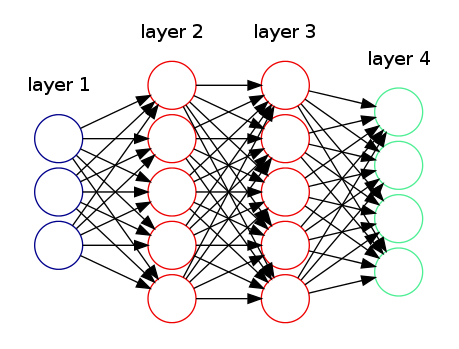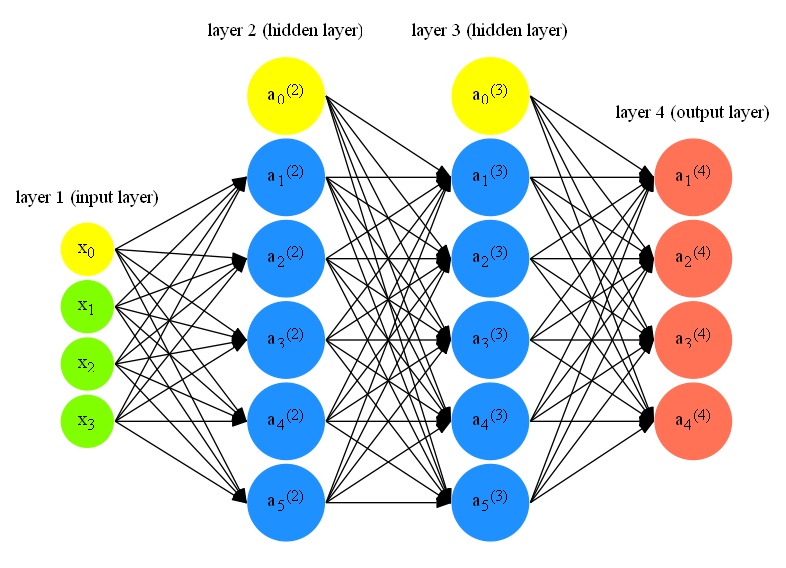# Draw a Neural Network through Graphviz

## Preface

Graphviz is a language (called DOT) and a set of tools to automatically generate graphs. It is widely used by researchers to do visualizations in papers. Essentially, you just need to provide a textual descritption of the graph regarding its topological structure (i.e. what nodes are, how they are connected, etc) and Graphviz will figure out the layout of the image by itself. Usually, the generated layout works out well but quite often, like this post mentioned, can be a "finicky beast". So, I decide to share some tips I learned about Graphviz.

Specifically, in this post, I'll demonstrate how we can draw the Neural Network shown in the last post and use this as an example to show some tricks in Graphviz to tweak the layout 1. Let's get started!

## Draw a neural network

If you do a quick search regarding "graphviz neural network example", you'll highly likely see the below picture:This is probably the simplest Graphviz demonstration on Neural Network. The code for this picture can be obtained here.

However, when I'm preparing my last post, I'm not quite satisified with the example above. I want to clearly label all the nodes in all layers and make distinction among feature input, bias term, hidden units, and output units. So, I decide to draw one on my own.

Here is the code that generates the picture below 2. Let me briefly highlights some key points in the code:``````rankdir = LR;
splines=false;
edge[style=invis];
``````
1. `rankdir=LR` makes the directed graphs drawn from left to right.
2. `splines=false` controls how the edges are represented and in this case, edges are drawn as line segments.
3. `edge[style=invis]` forces edges to become invisible. This is a common trick to tweak graphviz layout.
``````{
node [shape=circle, color=yellow, style=filled, fillcolor=yellow];
x0 [label=<x<sub>0</sub>>];
a02 [label=<a<sub>0</sub><sup>(2)</sup>>];
a03 [label=<a<sub>0</sub><sup>(3)</sup>>];
}
``````
1. `node[...]` sets the default node property: specify the node shape, node color. This node property will apply to three nodes: `x0`, `a02`, `a03`.
2. `x0 [label=<x<sub>0</sub>>]` specify the text label for node `x0`. The text for label is specified in HTML-like and this is how we write subscript and superscript in Graphviz.
3. `{...}` specifies the scope of the node property. This code chunk as a whole shows how we can specify several nodes at the once with the same node property 3.
``````{
rank=same;
x0->x1->x2->x3;
}
``````
1. `rank=same` is another trick I'll talk about later. This specifies what "layer" (or "rank" by official term) a set of nodes belongs. You can read the official doc for the details.
2. `x0->...->x3` specifies the relative position of the four nodes. Since the graph is arranged from left to right (indicate by `rankdir = LR`), then the "layer" is vertical. Then by `x0->...->x3`, the first node will be `x0`, followed by `x1`, and so on. Also, we have `edge[style=invis]` and this will hide the edges among these four nodes. This is how we draw the NN layers.
``````a02->a03;
``````
1. This line is used to prevent tilting of the graph. As you can see, we specify how the nodes should be arranged in a layer but we don't much constraint on how the layers should be positioned except `rankdir=LR`, which says layers should be ordered from left to right. `a02->a03` says layer with `a02` should be lined up with layer with `a03`.
``````l0 [shape=plaintext, label="layer 1 (input layer)"];
l0->x0;
{rank=same; l0;x0};
``````
1. This code chunk is how we add label text to each layer. As you can see we use another node `l0` with shape `plaintext`, which says `l0` is just a text message. Then we connect it with the first node of layer 1 `x0`, which serves as attaching the text to the layer 1.
``````edge[style=solid, tailport=e, headport=w];
``````
1. We specify the edge style again. This will only affect the edges after this setup not before. One small trick here is `tailport=e, headport=w`. This will let all the edges point to the same position.
``````{x0; x1; x2; x3} -> {a12;a22;a32;a42;a52};
{a02;a12;a22;a32;a42;a52} -> {a13;a23;a33;a43;a53};
{a03;a13;a23;a33;a43;a53} -> {O1,O2,O3,O4};
``````
1. This code chunk is how we actually draw the edges. In the simple example above, it explicitly draws the edges between two nodes. It is quite pain to do. Above code chunk provides a simpler way to achieve the same purpose.

## Graphviz tricks

From our NN drawing example, there are two recurring tricks when we tweak Graphviz picture layout:

• Invisible nodes/edges
• Rank constraints

### Invisible nodes/edges

In the above example, we use invisible edges to specify the ordering of nodes within each NN layer. In addition, we use node with `plaintext` shape to specify the text label in the layer.

Usually, we use invisible edges to specify what nodes should line up and sometimes we use invisible nodes to take up space to keep the graph in a specific structure. This SO post demonstrates how we can use invisible nodes and edges in combination to create a fancy picture. This SO post is another example to show how to use "invisible edges" (it uses another trick called `group` attribute).

### Rank constraints

If you check official doc, here is what rank does:

Rank constraints on the nodes in a subgraph. If rank="same", all nodes are placed on the same rank. If rank="min", all nodes are placed on the minimum rank. If rank="source", all nodes are placed on the minimum rank, and the only nodes on the minimum rank belong to some subgraph whose rank attribute is "source" or "min". Analogous criteria hold for rank="max" and rank="sink". (Note: the minimum rank is topmost or leftmost, and the maximum rank is bottommost or rightmost.)

Let's demonstrate this description with a simple example 4:

``````digraph G
{
{rank=source; a->b;}
{rank=same; c->d;}
}
``````

This example gives a graph with two rows. `a->b` is above `c->d`. However, if I change `{rank=source; a->b;}` to `{rank=min; a->b;}`, we'll end up with one row: `a->b` will be to the left of `c->d`. This is due to the difference between `min` and `source`: `min` allows other subgraphs in the minimum rank. However, `source` only allows other subgraphs of `min` or `source` to be on the minimum rank (we have `same` in this case).

`sink` and `max` works similarly. For instance, the below example gives a picture with `c->d` at the top and `a->b` at the bottom:

``````digraph G{
{rank=sink; a->b;}
{rank=same; c->d;}
}
``````

1. Of course, Graphviz is not the only tool that can produce beautiful pictures. TikZ is another popular tool. You can check out its NN example for comparison.

2. Technically, the code used to generate the blog NN picture is this one but the code I explained above is much more concise.

3. Check out this SO post for more examples on grouping nodes with the same attributes.

4. The example is adapted from this SO post